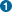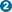## Name

FORCE — Force calculation for a Cartesian frequency analysis requested.

## Synopsis

` FORCE `

## Description

A frequency analysis in Cartesian coordinates is performed on the system. This is accomplished by computing the Hessian (that is, the matrix in millidynes per Angstrom of second derivatives of the energy with respect to displacement of all pairs of atoms in x, y, and z). When the Hessian is diagonalized, the force constants for the molecule are generated and a force matrix, appropriately weighted for isotopic masses, is calculated. The force matrix is then used to calculate the vibrational frequencies and thermodynamic properties. Before a FORCE calculation is performed, the gradient norm (gnorm) is computed for the input geometry, and if this value is larger than 3.00, the computation is halted. (Using LET will override this behavior and allow FORCE to proceed.) When one needs to characterize a stationary point but does not require all of the IR frequencies, LFORCE and HESSEI provide a low cost alternative to FORCE. See Chapter 4, Computational Procedures for a discussion of stationary point characterization.

Some care must be taken when characterizing stationary points on geometries with frozen variables. FORCE causes all geometric variables to be unfrozen and so may produce unexpected results. For example, an optimization with frozen variables followed by a FORCE calculation may fail because the constrained extrema may not correspond to a genuine extrema once the variables are unfrozen. The FORCE calculation can be made to proceed anyways by using LET, however, the resulting frequencies may not be meaningful. Alternatively, specifying LFORCE (instead of FORCE) will compute a few lowest Hessian eigenvalues and corresponding IR frequencies on the unconstrained geometry, without regard to the gradient norm. A third alternative is to use HESSEI, which computes the lowest Hessian eigenvalues (similar to LFORCE) but does so on the constrained geometry (unlike FORCE and LFORCE).

FORC

none

none

## Examples:

### Input File (`properties/prop_thermo_trans.dat`) :

```  am1 rhf singlet t=auto truste force rot=2 trans=2 print=1p-xylene
Thermo with TRANS=2 to remove internal rotation of methylsC              0.000000  0    0.000000  0    0.000000  0    0    0    0
C              1.395052  1    0.000000  0    0.000000  0    1    0    0
C              1.396919  1  120.474038  1    0.000000  0    2    1    0
C              1.396919  1  119.051836  1   -0.106881  1    3    2    1
C              1.395052  1  120.474038  1    0.106881  1    4    3    2
C              1.396919  1  120.474038  1    0.108409  1    1    2    3
H              1.100167  1  119.542900  1  179.980911  1    1    6    5
H              1.100167  1  119.983003  1 -179.979770  1    2    1    6
H              1.100167  1  119.542900  1 -179.980911  1    4    3    2
H              1.100167  1  119.983003  1  179.979770  1    5    4    3
C              1.480664  1  120.472114  1 -179.388508  1    3    2    1
H              1.119254  1  109.937276  1   89.635686  1   11    3    2
H              1.116344  1  111.043245  1 -149.505323  1   11    3    2
H              1.116344  1  111.043245  1  -31.223306  1   11    3    2
C              1.480664  1  120.472114  1 -179.388508  1    6    1    2
H              1.116344  1  111.043245  1 -149.505323  1   15    6    1
H              1.116344  1  111.043245  1  -31.223306  1   15    6    1
H              1.119254  1  109.937276  1   89.635686  1   15    6    1
0              0.000000  0    0.000000  0    0.000000  0    0    0    0
```
 The keyword FORCE calls for a frequency calculation (in Cartesians) along with the generation of thermodynamic data. In order to obtain correct results for the thermodynamic energy partition, the symmetry number for the point group must be given. The TS’s point group is C2h, requiring ROT=2. The keyword TRANS=2 is optional as the new AMPAC automatically accounts for the presence of two negative eigenvalues This geometry was pre-optimized. If the geometry is not pre-optimized, then one of AMPAC’s geometry optimizers (energy minimizers: TRUSTE, EF, NEWTON, BFGS, DFP; gradient minimizers: TRUSTG, TS, or LTRD) should be specified along with FORCE, so that the geometry will be optimized just prior to computing frequencies.